# Efficient synchronisation of state-based CRDTs

Efficient synchronisation of state-based CRDTs Enes et al., arXiv’18

CRDTs are a great example of consistency as logical monotonicity. They come in two main variations:

• operation-based CRDTs send operations to remote replicas using a reliable dissemination layer with exactly-once causal delivery. (If operations are idempotent then at-least-once is ok too).
• state-based CRDTs exchange information about the resulting state of the data structure (not the operations that led to the state being what it is). In the original form the full-state is sent each time. State-based CRDTs can tolerate dropped, duplicated, and re-ordered messages.

State-based CRDTs look attractive therefore, but over time as the state grows sending the full state every time quickly becomes expensive. That’s where Delta-based CRDTs come in. These send only the delta to the state needed to reconstruct the full state.

Delta-based CRDTs… define delta-mutators that return a delta ($\delta$ ), typically much smaller than the full state of the replica, to be merged with the local state. The same$\delta$ is also added to an outbound$\delta-$buffer, to be periodically propagated to remote replicas. Delta-based CRDTs have been adopted in industry as part of the Akka Distributed Data framework and IPFS.

So far so good, but the analysis in this paper reveals an unexpected issue: when updates are frequent enough that concurrent update operations are occurring between synchronisation points, delta-propagation algorithms perform no-better than sending the full state.

You can see the effect in the following chart: delta-based propagation is transmitting pretty much the same amount of data as full state-based.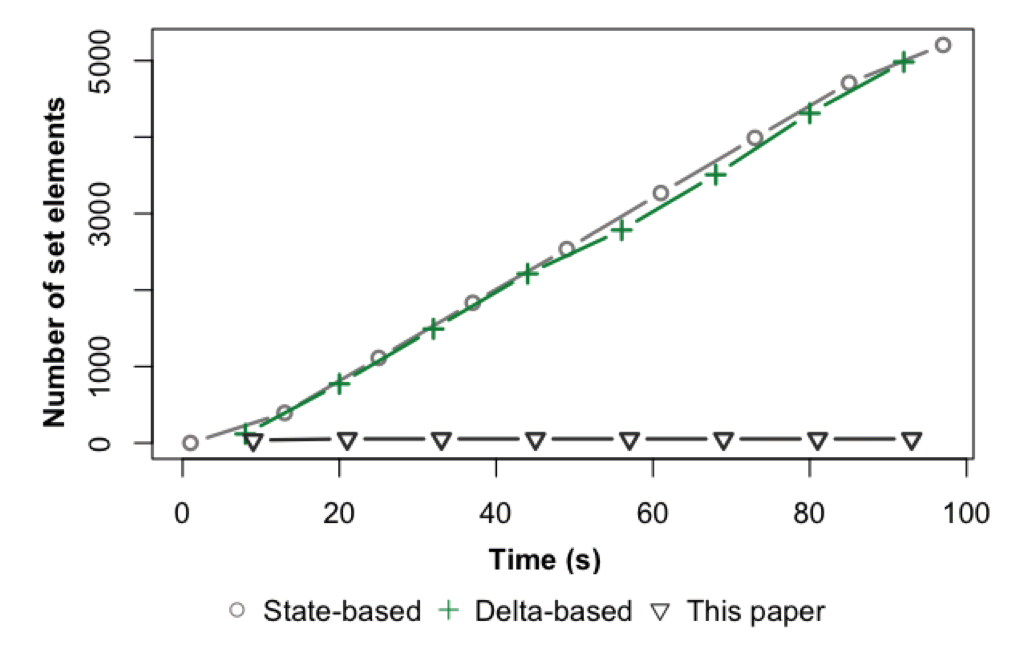Moreover, due to the overheads of computing deltas etc., delta-propagation is also consuming a lot more CPU to do so.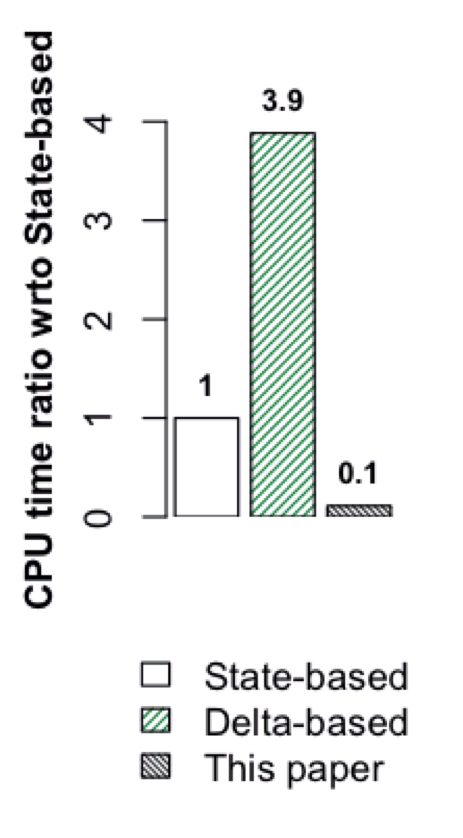I’m sure you’ve also spotted in those two figures the additional data series for ‘this paper.’ Two key enhancements to the delta-propagation algorithm are introduced which greatly reduce both the amount of data transmitted and the CPU (and memory) overheads.

### Some example state-based CRDTs

To bring all this to life we need a couple of examples. The paper uses grow-only CRDT counters and grow-only sets. State-based CRDTs are based upon a join semi-lattice,$\mathcal{L}$, a set of mutators that update that state, and a binary join operator$\sqcup$ that derives the least upper bound for any two elements of$\mathcal{L}$.

For a grow-only counter, we keep track of one (sub) counter per replica. The inc operation increments the replica-local counter, the join operator takes the higher of the two replica-local counter values for each replica, and the overall counter value is produced by summing the replica-local counters.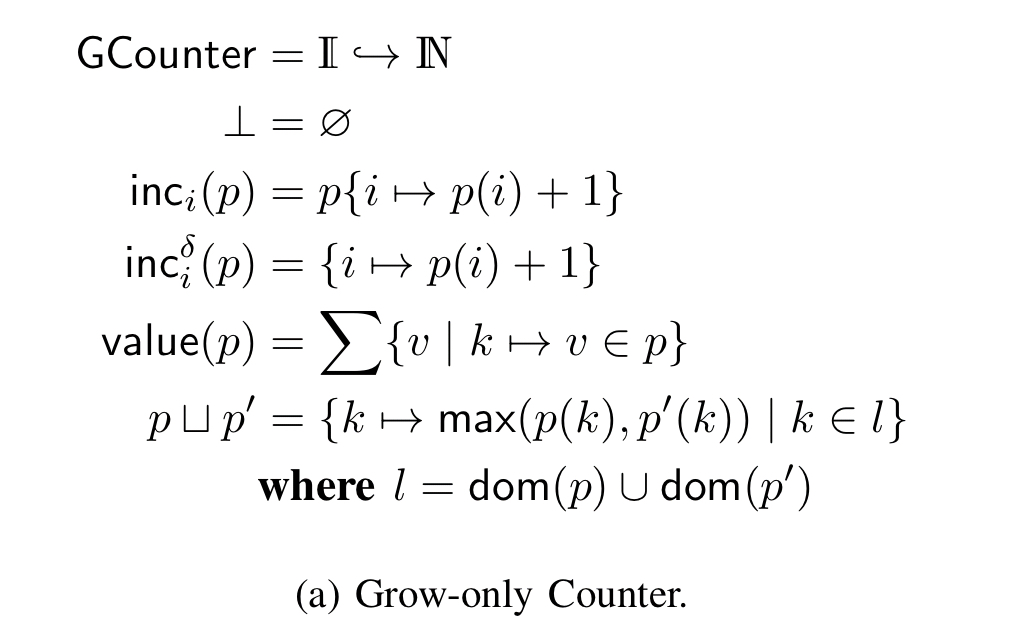For a grow-only set (GSet) we just add elements to the set and the join operator is a set union.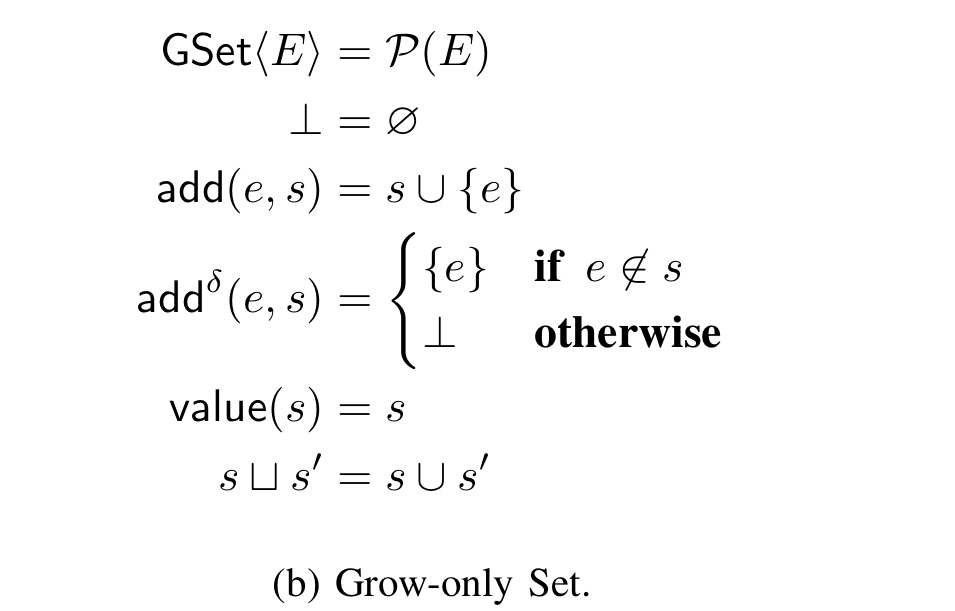Hasse diagrams can be used to show the evolution of the lattice. For example: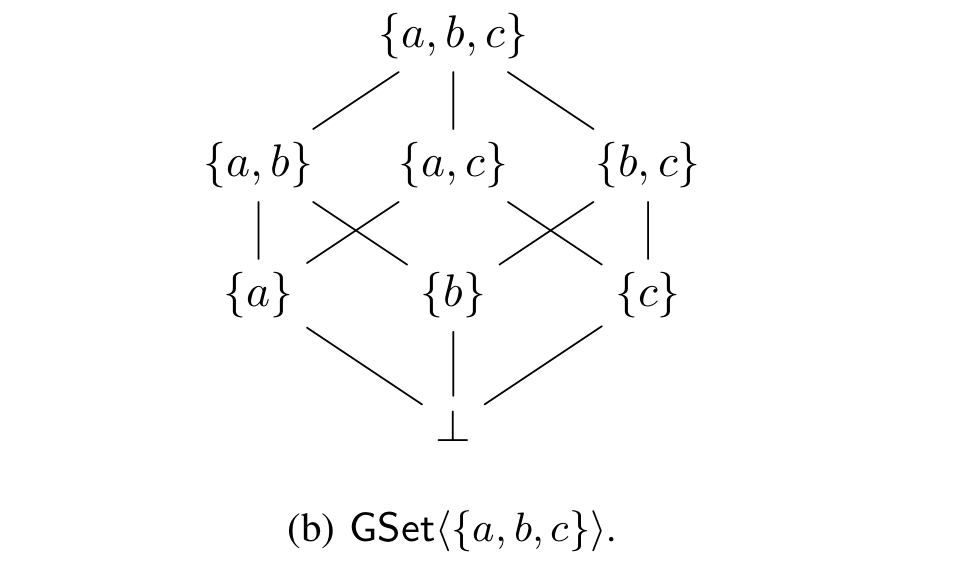### Spotting inefficiencies

Let’s look at one particular evolution of a GSet in a scenario with two replicas, A and B. In the diagram below,$\bullet$ marks a point in time where synchronisation occurs. For example, at$\bullet_1$, replica B sends the delta$\{b\}$ to replica A. A has also received a local update. At$\bullet_2$, replica A sends all updates it has received since the last time it propagated changes back to B. This is the set$\{a, b\}$. Meanwhile replica B has added$c$, and at$\bullet_3$ it sends all of its changes since$\bullet_1$ back to A.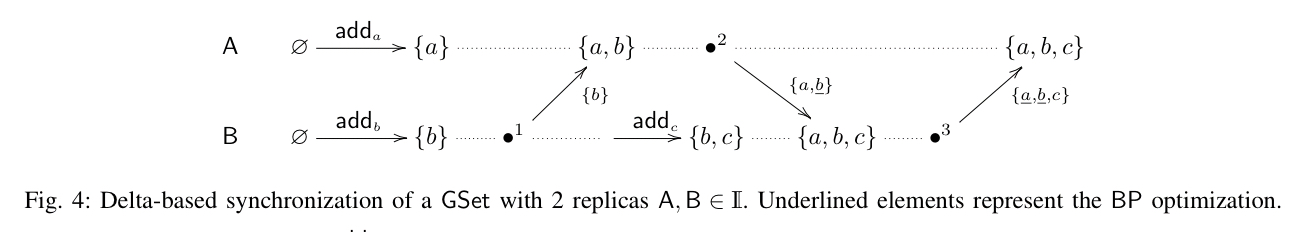It’s easy to spot some redundancy in transmission here. At$\bullet_1$, B sends$\{b\}$ to A, and at$\bullet_2$, A is including$\{b\}$ in the update it sends back to _A_ again. All of the underlined elements in the figure above are redundant transmissions of this type.

So it’s not exactly rocket science…

> … by simply tracking the origin of each$\delta$-group in the$\delta$-buffer, replicas can avoid back-propagation of$\delta$-groups.

The BP optimisation in effect says “don’t send an update back to the replica that told you about it in the first place!” In order to do that, we’ll need some extra book-keeping to remember where updates have come from. The evaluation shows this is worth it.

Here’s another example trace that shows a related issue: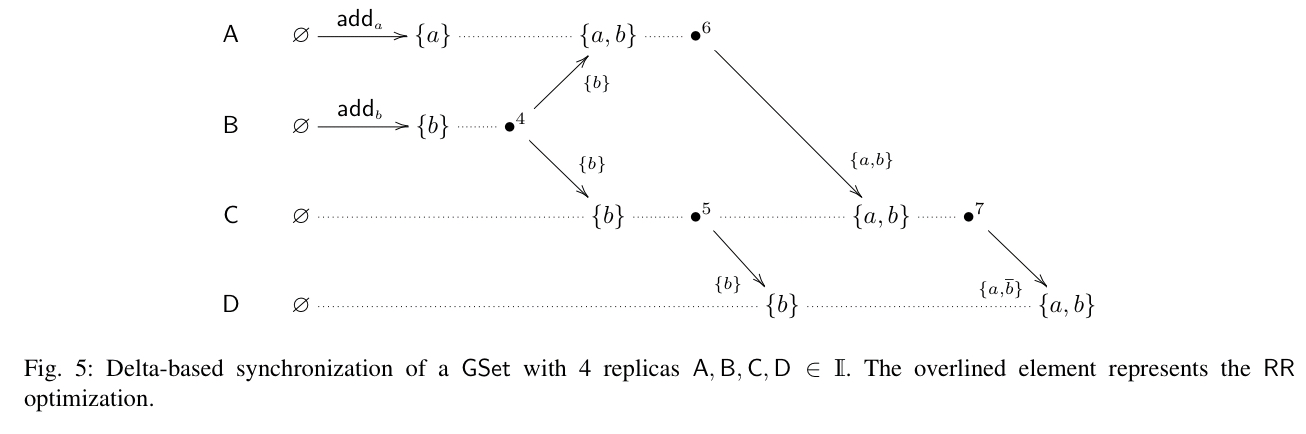Here we see that at$\bullet_5$, C is sending delta$\{b\}$ to D. Then C receives an update$\{a, b\}$ from A at$\bullet_6$. At$\bullet_7$ the update$\{a, b\}$ is propagated from C to D, with the result that C has redundantly sent$\{b\}$ to D in this second transmission. The RR optimisation removes this redundant transfer, we should…

> … remove redundant state in received$\delta$-groups (RR) before adding them to the delta buffer.

And that’s it! Don’t send an update back to the replica that sent it to you, and don’t send the same update to the same replica more than once.

### A revised delta-based synchronisation algorithm

Now, those ideas are formalised using the notion of a join-irreducible state. A join irreducible state$x$ is one that can’t be reconstructed from a join of some finite set of states not containing$x$. So the set$\{a\}$ is join irreducible, but the set$\{a, b\}$ is not because we can construct it by joining$\{a\}$ and$\{b\}$. Every CRDT has a unique irredundant decomposition of a state into a set of join irreducible states. For the GSet for example, these are just the sets containing individual elements, and for the GCounter they are the individual replica-local counters. We can use this decomposition to find the minimum delta,$\Delta(a,b)$ between two states$a$ and$b$.

Here’s the classic delta-based synchronization algorithm for replica$i$, updated to include the BP and RR optimisations. Highlighted lines show the changes.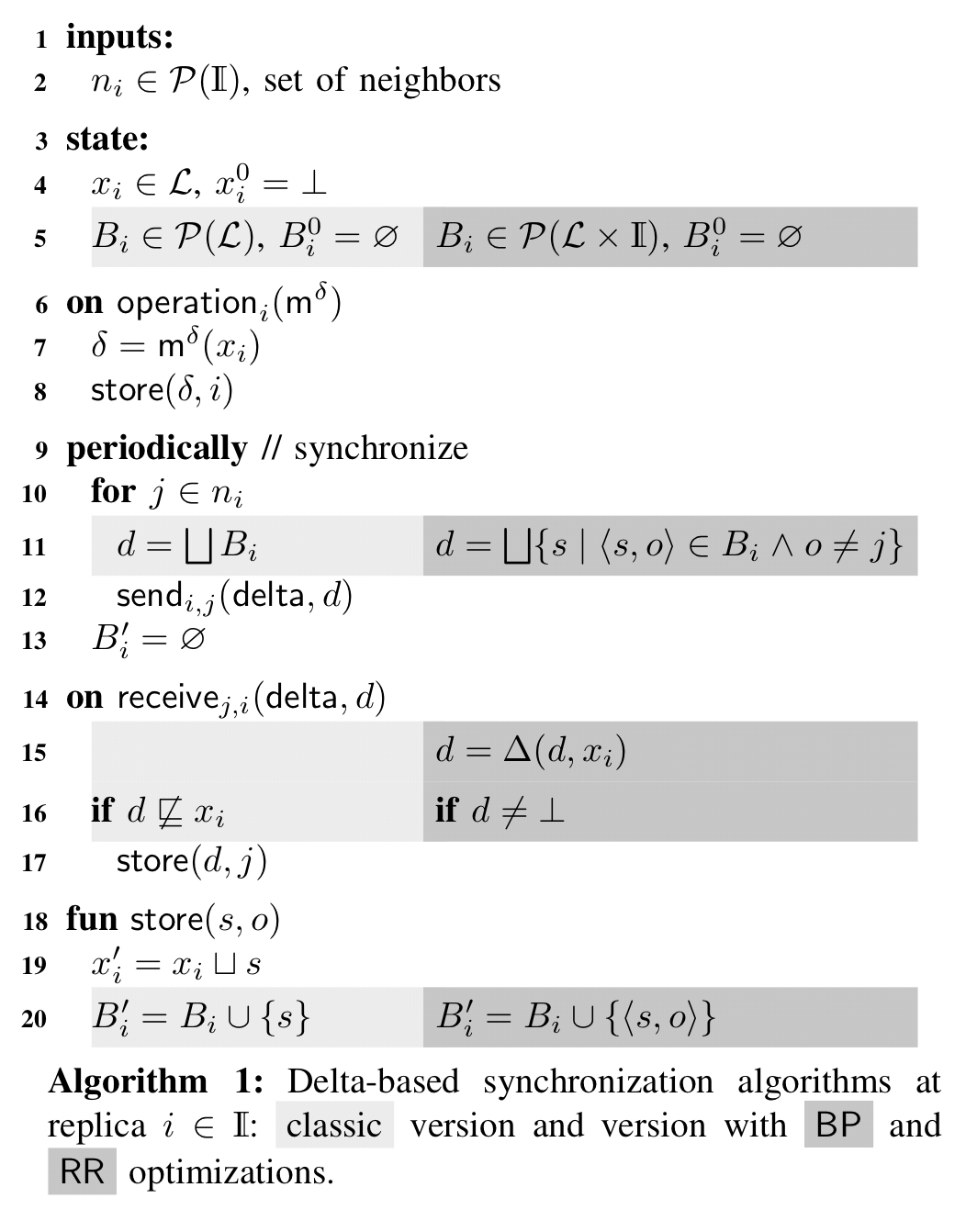For BP, each$\delta$-buffer is tagged with its origin at line 5, so that we can filter out redundant deltas when sending updates in line 11. For RR we extract the minimal delta in line 15.

### Evaluation

The evaluation takes place over two different network topologies. Both have 15 nodes, but one is cyclic (mesh) and one is acyclic (tree).Each node performs an update and synchronises with its neighbours once per second, as per the table below.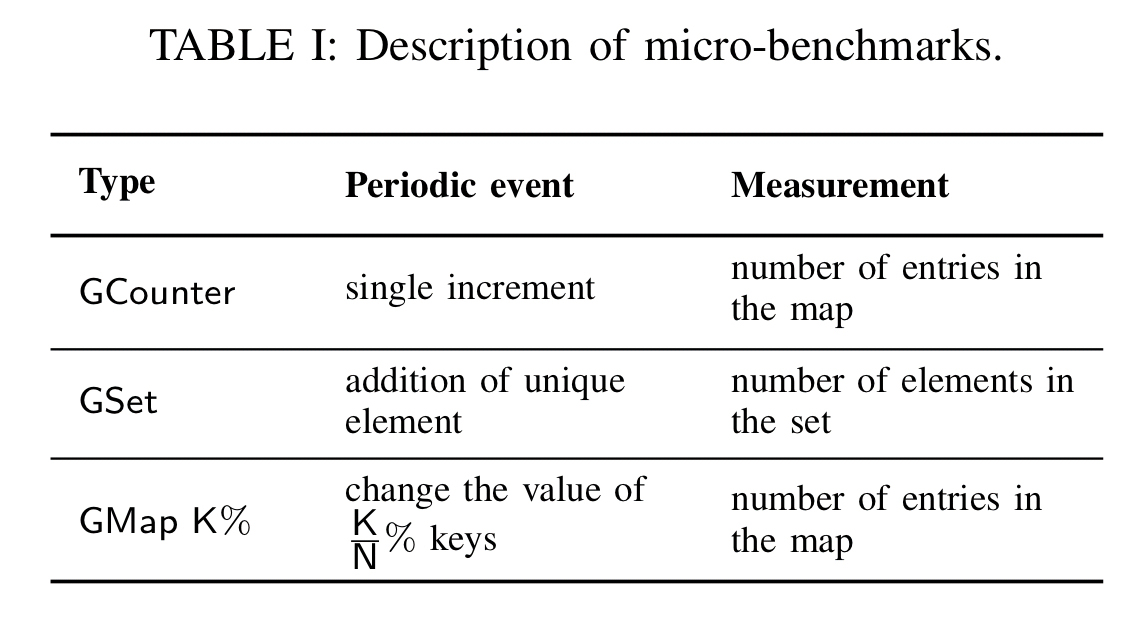Comparisons are made to vanilla delta state, delta state with each optimisation individually, delta state with both optimisations, and also state-based CRDTs (full state exchange), operation-based CRDTs, and CRDTs using the Scuttlebut anti-entropy protocol.

Here’s an analysis of the amount of data transmitted by GSet and GCounter in the tree and mesh topologies.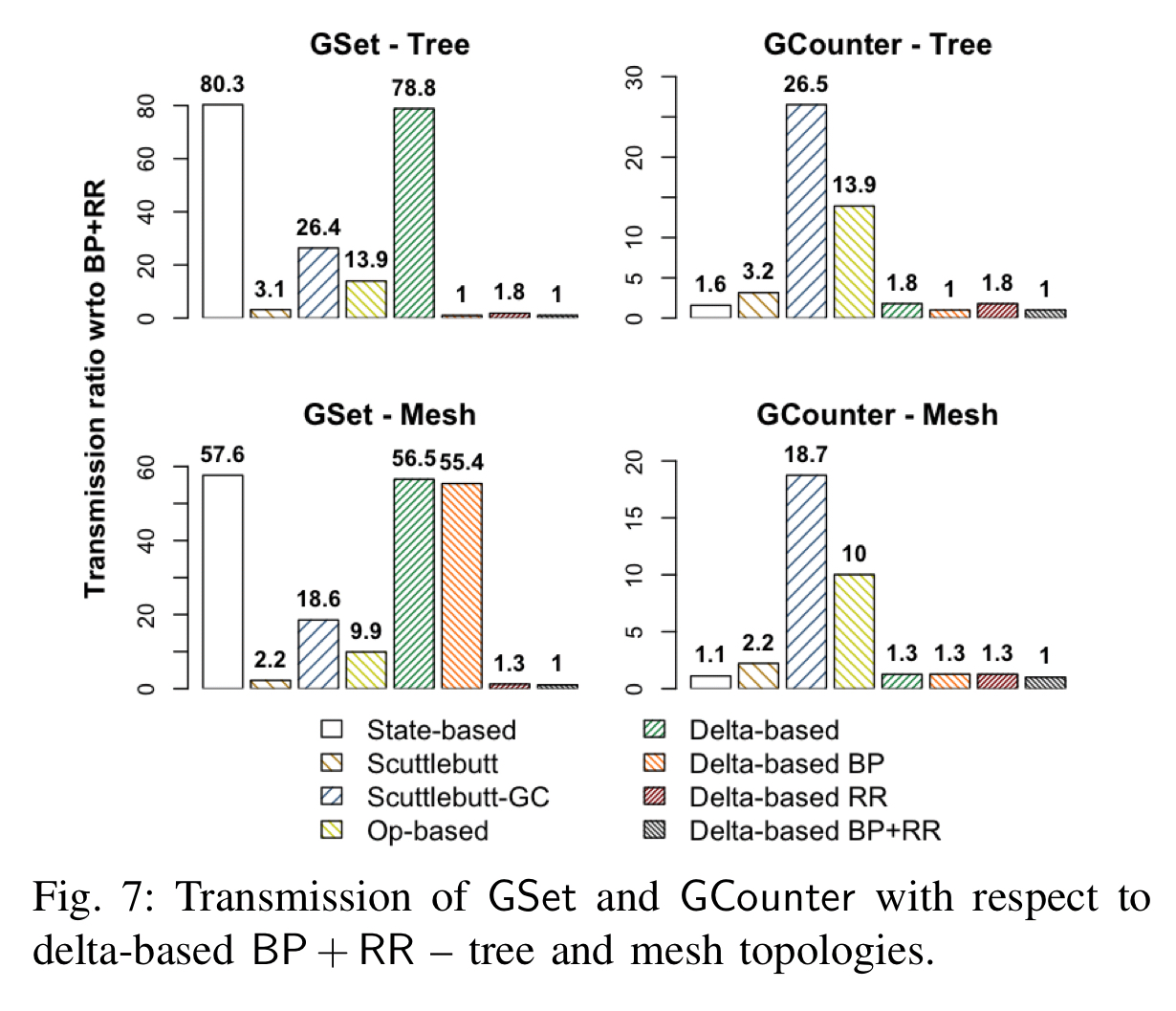The first observation is that the classic delta-based synchronization presents almost no improvement, when compared to state-based synchronization.

In the tree topology, BP alone is enough to give good results, whereas the mesh topology benefits from RR.

Compared to Scuttlebut and operation-based CRDTs, delta-based CRDTs with both BP and RR have a much lower metadata overhead. The overhead can be as high as 75% with the former group, whereas the optimised delta-based algorithm can get this as low as 7.7%.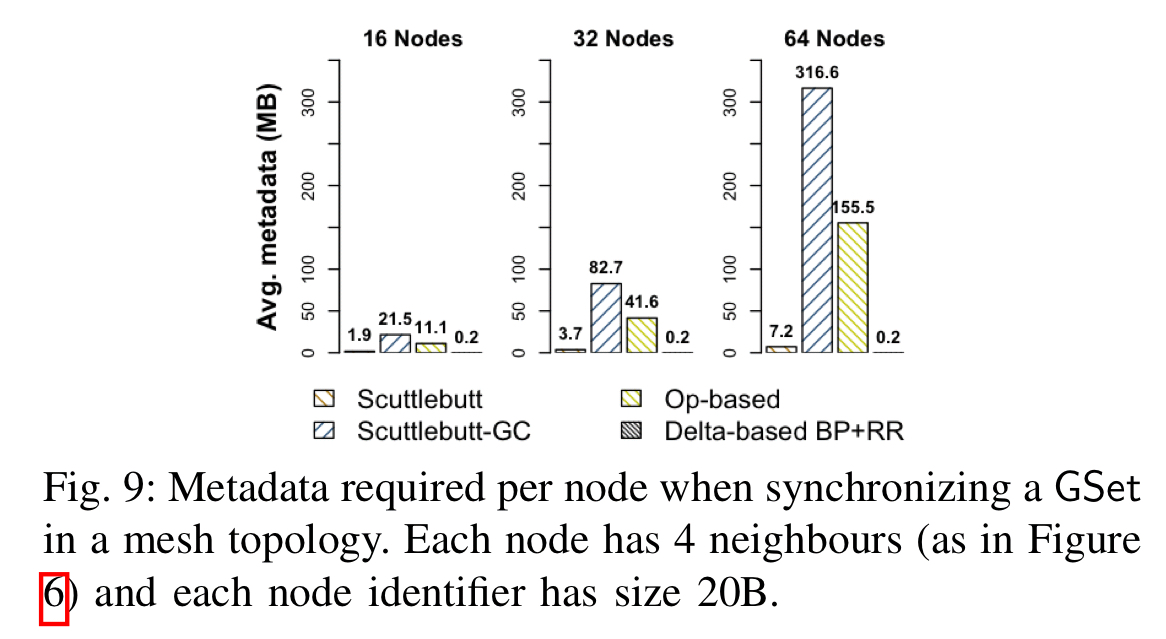When used in the Retwis twitter clone for a moderate-to-high contention workload, the BP + RR optimisations can reduce CPU overhead by up to 7.9x compared to the classic delta-based algorithm.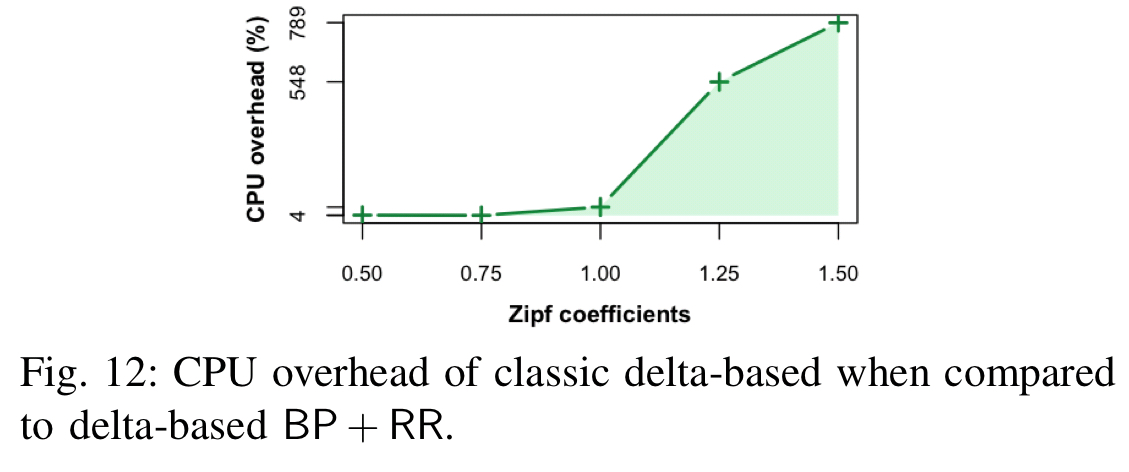State-based CRDT solutions quickly become prohibitive in practice, if there is no support for treatment of small incremental state deltas. In this paper we advance the foundation of state-based CRDTs by introducing minimal deltas that precisely track state changes.

De-dup FTW!